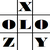anything in Excel...

It's Excel-o-lozy...

### Excel Formula to convert Date from to valid date format like YYYYMMDD to DDMMYYYY under formulaFix by Excelolozy.

www.Excelolozy.com - It's now easy to Convert YYYYMMDD to DDMMYYYY i.e. the Date from Raw text format to recognisable and valid Date format.

Following is the Formula :

formula is "ConvertDate"

=ConvertDate(Ref,OldFormat)

The formula "ConvertDate" is available within tool named "formulaFix".

"formulaFix" is a "Formula Fixing Tool" that provides various formulas that don't exist in Excel.

Examples of formula "ConvertDate" are as follows :

 Raw Date Old Format Formula Converted Date result Remarks 20121013 if omitted =convertdate(A2,) Saturday, October 13, 2012 YYYYMMDD is taken by default if omitted in the formula. 13102012 DDMMYYYY =convertdate(A3,B3) Saturday, October 13, 2012 Any type of OLD FORMAT can be converted to any type of NEW FORMAT. 322012 DMYYYY =convertdate(A4,B4) Friday, February 03, 2012 2313 DMYY =convertdate(A5,B5) Saturday, March 02, 2013 2032013 DMMYYYY =convertdate(A6,B6) Saturday, March 02, 2013 2013 03 2 YYYY MM D =convertdate(A7,B7) Saturday, March 02, 2013 20130302 YYYYMMDD =convertdate(A8,B8) Saturday, March 02, 2013 03-02-2013 MM-DD-YYYY =convertdate(A9,B9) Saturday, March 02, 2013 Any character of OLD FORMAT can be omitted by placing any character in NEW FORMAT. 13aaa3aaa02 YYxxxMxxxDD =convertdate(A10,B10) Saturday, March 02, 2013 13bbb3bbb02 YYxxxMxxxDD =convertdate(A11,B11) Saturday, March 02, 2013

To see practical application of above table do as follows :